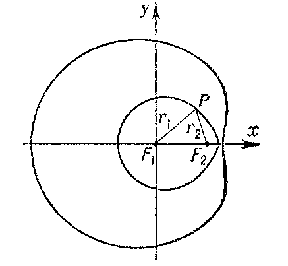# Descartes oval

A plane curve for which the distances $r_1$ and $r_2$ between any point $P$ of the curve and two fixed points $F_1$ and $F_2$ (the foci) are related by the non-homogeneous linear equation

$$r_1+mr_2=a.$$

A Descartes oval may be defined by means of the homogeneous linear equation

$$r_1+mr_2+nr_3=0,$$

where $r_3$ is the distance to the third focus $F_3$ located on the straight line $F_1F_2$. In the general case, a Descartes oval consists of two closed curves, one enclosing the other (see Fig.). The equation of a Descartes oval in Cartesian coordinates is

$$\sqrt{x^2+y^2}+m\sqrt{(x-d)^2+y^2}=a,$$

where $d$ is the length of the segment $F_1F_2$. If $m=1$ and $a>d$, the Descartes oval is an ellipse; if $m=-1$ and $a<d$, it is a hyperbola; if $m=a/d$, it is a Pascal limaçon. First studied by R. Descartes in the context of problems of optics .Figure: d031340a

How to Cite This Entry:
Descartes oval. Encyclopedia of Mathematics. URL: http://encyclopediaofmath.org/index.php?title=Descartes_oval&oldid=31380
This article was adapted from an original article by E.V. Shikin (originator), which appeared in Encyclopedia of Mathematics - ISBN 1402006098. See original article